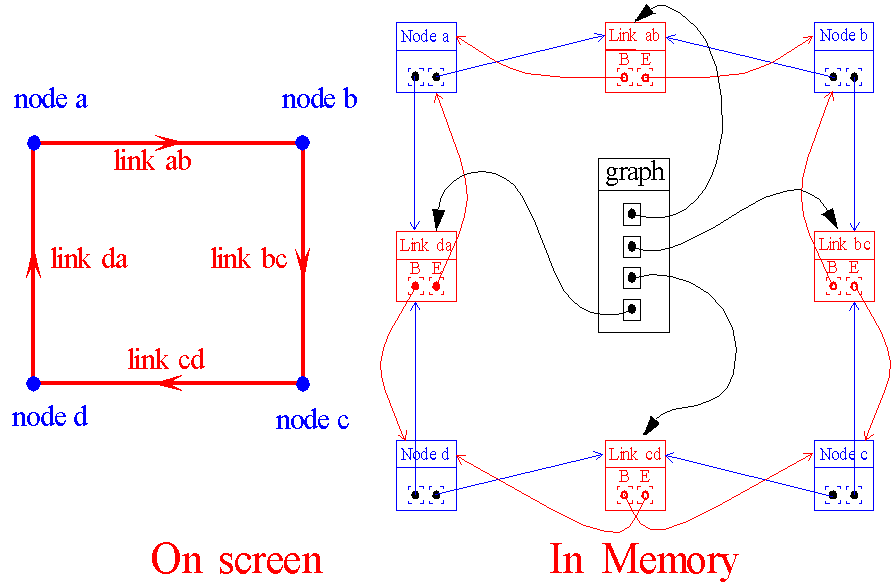Datastructure

# terminology

•      polygon = list of vertexes used to represent a closed polygon
•      graph= list of links, represents the segments of the original polygons.
•      graphlist = list of graphs
•      link = connection between two nodes (represents one segment of the original polygon)
•      node = connection between several links, has a (x,y) position

# graphs

The boolean operation uses an internal data structure that is based on graphs. The original polygons are first converted into graphs, each polygon
becomes one graph. A graph is in principal a list of segments/links, where each link has two pointers. One pointer to the beginnode of the segment
and one to the endnode. Several links can share the same node. At first each node in the graph will be referenced by two links, the reason is that
the original polygon is always a closed shape. All the links in a graph get a unique number to tell that they belong to a certain polygon.
When intersections between graphs have been calculated at the intersection points, new nodes are created that can have many links
pointing to it, but at least 4 links. In Graph structure. the memory structure of the graph that represent a polygon containing 4 vertexes is
shown. In Intersection of graph links. is shown what happens when an intersection point is found. At the intersection point of the two
intersecting links, a new node is inserted and the original links are split into two parts.
For the intersection process the seperate graphs are merged into one big graph. After intersection of several graphs, the original polygons can still
be distinguished by following the unique number of a specific link.
When all intersections are known, a flag is set for the right and left side of each link to tell if it belongs to groupA or groupB enclosed areas.
Based on this information the proper result for a given boolean operation can be extracted.

## Graph structure